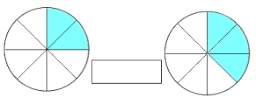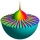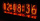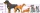# Sum of fractions

What is the sum of 2/3+3/5?

f =  1.2667

### Step-by-step explanation:

$f=\frac{2}{3}+\frac{3}{5}=\frac{19}{15}=1\frac{4}{15}=1.2667$Did you find an error or inaccuracy? Feel free to write us. Thank you!Dr Math
For more detailed step-by-step solution see our fraction calculator:

https://www.hackmath.net/en/calculator/fraction?input=2%2F3%2B3%2F5&submit=CalculateMath student
-18 divided by 3Tips to related online calculators
Need help to calculate sum, simplify or multiply fractions? Try our fraction calculator.

## Related math problems and questions:

• Simplify 3Simplify mixed numerals expression: 8 1/4- 3 2/5 - (2 1/3 - 1/4) Show your solution.
• Mixed numbersRewrite mixed numbers, so the fractions have the same denominator: 5 1/5 - 2 2/3
• Fraction + eqSolve following simple equation with fractions: -5/6(8+5b) = 75 + 5/3b
• Turtles 2A box turtle hibernates in the sand at 11 5/8. A spotted turtle hibernates at 11 16/25 feet. Which turtle is deeper? Write answer as number 1 or 2.
• Eq2 2Solve following equation with quadratic members and rational function: (x2+1)/(x-4) + (x2-1)/(x+3) = 23
• Evaluate 5Evaluate expression x2−7x+12x−4 when x=−1
• Linear combination of complexIf z1=5+3i and z2=4-2i, write the following in the form a+bi a) 4z1+6z2 b) z1*z2The heights of five starters on redwood high’s basketball team are 5’11”, 6’3”, 6’6”, 6’2” and 6’. The average of height of these players is?
• Solve 2Solve integer equation: a +b+c =30 a, b, c = can be odd natural number from this set (1,3,5,7,9,11,13,15)
• LCD two fractionsWhat is the least common denominator of 11/15 and 12/19?
• Sum of mixedThe sum of two mixed numbers is 15½. One of the number is 5 ¾, what is the other?
• Watching TVOne evening 2/3 of students watch TV. Of those students, 3/8 watched a reality show. Of the students that watched the show, 1/4 of them recorded it. What fraction of the students watched and recorded reality tv.
• EmilyEmily had 20 minutes to do a three-problem quiz. She spent 11 3/4 minutes on question A and 5 1/2 minutes on question B. How much time did she have left for question C?
• Prime factorsWrite 98 as a product of prime factors
• BoatsThree-quarters of boats are white, 1/7 are blue and 9 are red. How many boats do we have?3 3/4 + 2 3/5 + 5 1/2 Show your solution.Year; money spent on advertising; profit (three values each row) 2008 2 12 2009 5 20 2010 7 25 2011 11 26 2012 15 40 1. draw a scatter diagram depicting the data. 2. calculate the Pearson's correlation coefficient. 3. determine the linear regression equat